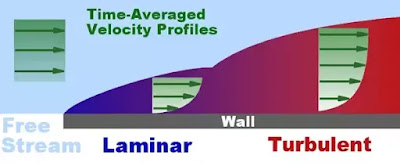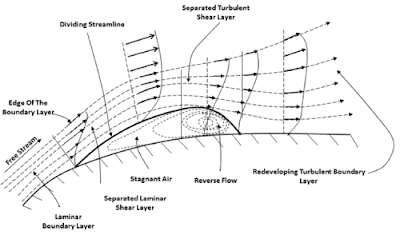# Boundary Layer - Explained

The boundary layer (BL) is a relatively thin layer of fluid close to the surface of a body that is immersed in a flow of fluid.The grey-part in the image above shows the BL, where the velocity in the main-flow (freestream) direction increases from the boundary surface to the free-stream boundary. (For the most practical considerations the edge of the boundary layer is taken to be the line where
${u}_{y}=0.99{u}_{0}$
The important thing to note is that within the boundary layer the effects of viscosity are important. Therefore even if the freestream may have a high Reynolds Number and therefore be considered inviscid, the same is not true inside the BL.
However, the nature of the BL allows us to produce a highly simplified version of the infamous Navier Stokes (NS) equations and makes calculations significantly easier.
If $u$ is the streamwise velocity (as in the figure) and $v$ is the velocity normal to the wall, then the N-S Equations in 2 dimensions reduce to
$u\frac{\mathrm{\partial }u}{\mathrm{\partial }x}+v\frac{\mathrm{\partial }u}{\mathrm{\partial }y}=-\frac{1}{\rho }\frac{\mathrm{\partial }p}{\mathrm{\partial }x}+\nu \frac{{\mathrm{\partial }}^{2}u}{\mathrm{\partial }{y}^{2}}$
and
Since there is no pressure gradient in the y direction and therefore the pressure in the boundary layer at a certain station in the boundary layer is the same as the free-stream pressure above it.
Now boundary layers can be both laminar and turbulent and indeed, for most high-speed flows, the BL starts off as initially laminar before thickening to a point where it transitions to turbulence.The difference between the two is that the laminar boundary layer offers very little skin friction and the accompanying drag is considerably lessened. Therefore, it is in the interest of aircraft designers that flow over the aircraft wings should be laminar over most of the airfoil. This can be achieved using various airfoil shapes and one of the first aircraft to incorporate the laminar wing intentionally as part of the design was the iconic P-51 Mustang, where it contributed substantially to the aircraft’s superlative performance in terms of range.However, for low-speed flight, typical of UAVs and model aircraft, the laminar boundary layer seperates from the wing surface as soon as it encounters the adverse pressure gradient over the rear of the wing. This leads to flow-seperation leading to the formation of a laminar seperation bubble and a huge increase in pressure drag. In such cases the BL is “tripped” to turbulence deliberately and although this results in an increase in skin friction drag, the overall drag is reduced.